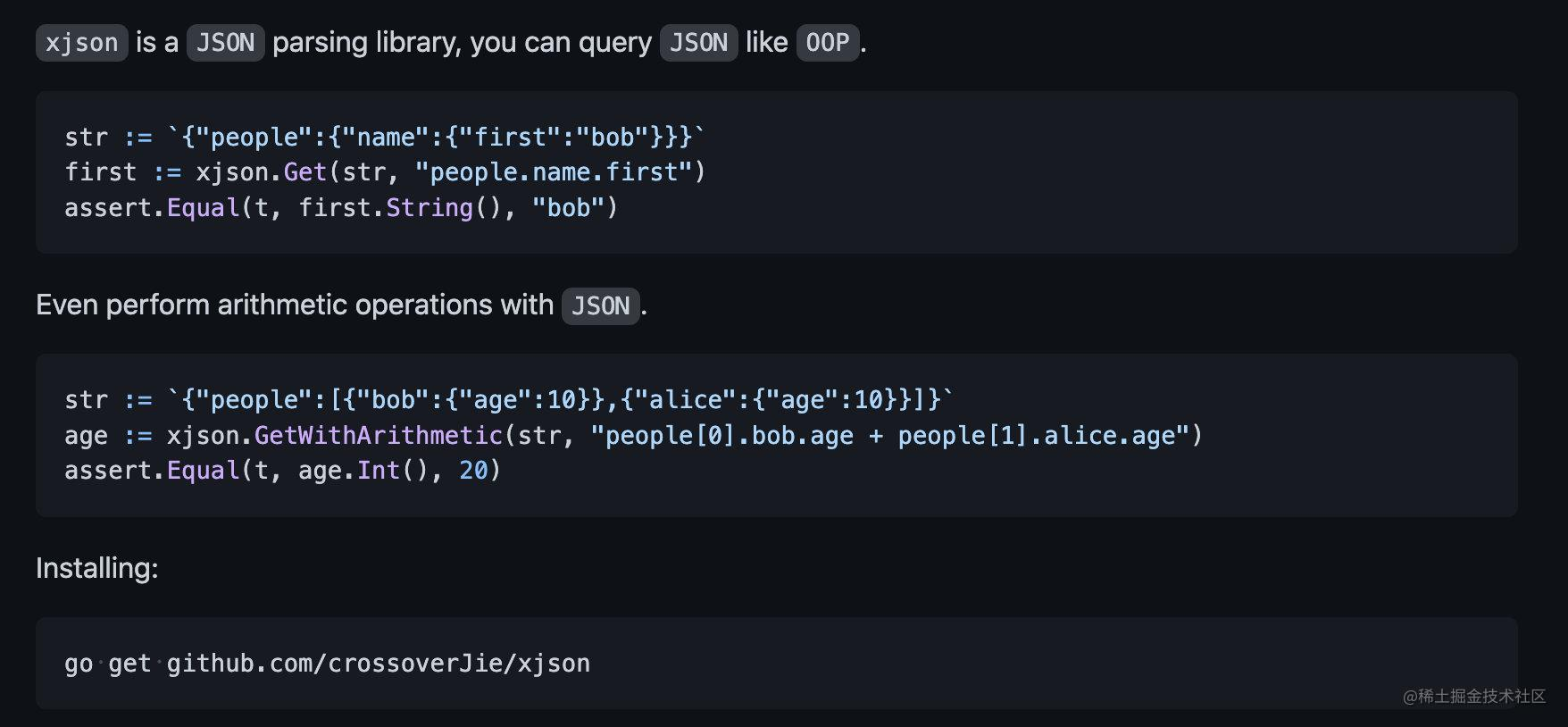# 如何实现一个 JSON 解析库# 前言1. 对转义字符的支持。
2. 性能优化，大约提升了30%⬆️。

## 转义字符

``````	str = `{"1a.b.[]":"b"}`
get = Get(str, "1a\\.b\\.\\[\\]")
assert.Equal(t, get.String(), "b")

str = `{".":"b"}`
get = Get(str, "\\.")
assert.Equal(t, get.String(), "b")

str = `{"a":"{\"a\":\"123\"}"}`
get = Get(str, "a")
fmt.Println(get)
assert.Equal(t, get.String(), "{\"a\":\"123\"}")
assert.Equal(t, Get(get.String(), "a").String(), "123")

str = `{"a":"{\"a\":[1,2]}"}`
get = Get(str, "a")
fmt.Println(get)
assert.Equal(t, get.String(), "{\"a\":[1,2]}")
assert.Equal(t, Get(get.String(), "a").Int(), 1)

## 性能优化

``````pkg: github.com/crossoverJie/xjson/benckmark
cpu: Intel(R) Core(TM) i7-9750H CPU @ 2.60GHz
BenchmarkDecode-12        	   14968	     77130 ns/op	   44959 B/op	    1546 allocs/op
PASS

------------------------------------
pkg: github.com/crossoverJie/xjson/benckmark
cpu: Intel(R) Core(TM) i7-9750H CPU @ 2.60GHz
BenchmarkDecode-12        	   19136	     62960 ns/op	   41593 B/op	    1407 allocs/op
PASS

# 实现四则运算

``````	json :=`{"alice":{"age":10},"bob":{"age":20},"tom":{"age":20}}`
query := "(alice.age+bob.age) * tom.age"
arithmetic := GetWithArithmetic(json, query)
assert.Equal(t, arithmetic.Int(), 600)

1. `json` 进行词法分析，得到一个四则运算的第一步 `token`
2. 基于该 `token` 流，生产出最终的四则运算表达式，比如 `(3+2)*5`
3. 调用四则运算处理器，拿到最终结果。